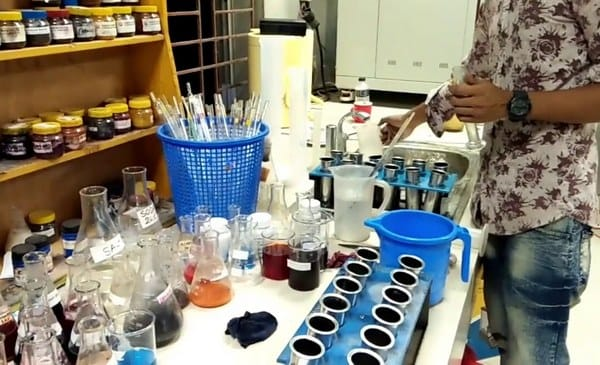# Dyeing Recipe Calculation in Textile Industry

Last Updated on 15/05/2022

Dyeing Recipe Calculation:
A lab dip is a sample of fabric that has been dyed as per requirements. When a dye house or color lab dyes a few small swatches of actual quality fabric or textile trim to find out what formula creates the best color match for that quality. Lab dips are very essential before dyeing bulk or even small lots of anything. In lab dip calculation some formulas are used. These formulas are described below.Dyeing Recipe Calculation Formula:

Dye = (Shade % * Weight of the fabric in gm) / Stock solution %

Or,

Required solution = WP / C

Where,
W = weight of fabric, yarn, or fiber
C = concentration of stock solution
CC = cubic centimeter.

For auxiliaries (chemicals) the formula is as below:

Required amount of solution (mls) = (g/l required * wt of substrate * LR) / (10 * concentration (%) of stock soln)

For addition of auxiliaries in solids form such as salt the formula is:
Salt in g/l = (Required amount (%) * Sample weight * LR) / 1000

Conversion formula from percentage to g/l is as below:
g/l = Required amount (%) * 10.

If alkali conc. Is given in Be. then the formula to calculate this in g/l is as follows :
Required amount of solution (mls) = (g/l required * wt of substrate * LR) / (10 * concentration (%) of stock soln)

Or ,

= (Required amount (%)* wt of substrate * LR) / (Concentration (%) of stock soln)

Or,

Required alkali soln in c.c. = (g/l required * wt of substrate * LR) / (10* conversion value from Be. to g/l of alkali)

Or ,

Required alkali soln in c.c. = (Required amount (%) * wt of substrate * LR) / conversion value from Be. to g/l of alkali

Example:
Suppose a lab deep of a fabric sample (1*1 rib) has to be formed with following dyes and chemicals:

Dyes:
1. Rema Blue RR = 1.122%
2. React Red KHW = 2.014%
3. React Yellow KHW = 1.486%

Salt = 70%
Soda Ash (conc.20%) = 5 g/l
Caustic Soda (38 Be) = 1.32%
L: R = 1:8
Sample Wt. = 5 gm
% Stock Soln = 1

Therefore, recipe calculation for dyes and auxiliaries in g/l will be as follows:

For dyes:

We know,
Dye  = (Shade % * Weight of the fabric in gm) / (Stock solution % )

For,
1. Rema Blue RR = (1.122*5)/1=5.61 g/l
2. React Red KHW = (2.014*5)/1= 10.07 g/l
3. React Yellow KHW = (1.486*5)/1= 7.43 g/l .

For auxiliaries:

We know,
Salt in g/l = (Required amount (%) * Sample weight * LR) /  1000
Required Salt = (70*5*8)/1000 = 2.8 gm.

For Soda ash (conc.20%):

We know ,
Required amount of solution (mls) = (g/l required * wt of substrate * LR) /  (10 * concentration (%) of stock soln)
.
.   . Required amount of soda ash in C.C. = (5*5*8) / (10*20) = 1.0

For Caustic soda (38 Be.):

We know ,
Required alkali soln in c.c. = (Required amount (%) * wt of substrate * LR ) / conversion value from Be. to g/l of alkali
.
.   . Required caustic soda = (1.32*5*8) / 441 = 0.12 c.c.

[since 38 °Be.NaOH = 441 gm NaOH 100% per 1lit NaOH soln]

Extra Water required:
= M:L – (required water to make soln of dyes and auxiliaries) = (5*8) – [(5.61+10.07+7.43) + (1.0+0.12) ]
= 40 – 24.112
= 15.77 (Salt is added in solid form)

Equipments of Recipe Section:

1. Microprocessor pH Meter (Hanna Instrument)
2. Digital pipette
3. Digital Weighting Meter with Glass Box (Explorer, USA)

You may also like: